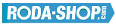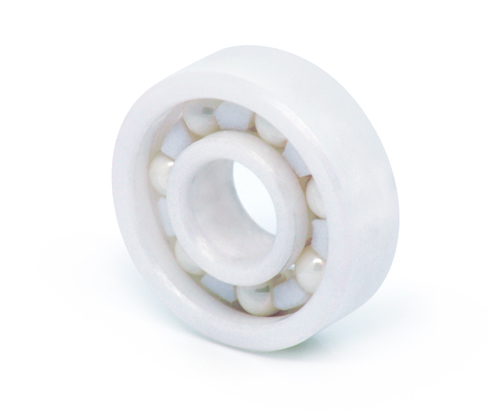# Hybrid and Ceramic BearingsManufacturer Product Image Item Name Price### 6002-CE-ZR02-PFTE

Solid Ceramic Bearings Ceramic Open(No Shields) Dimension d=15 Dimension D=32 Dimension B=9 Dinamic Load Rating=3360 Static Load Rating=1698 Weight=0.031gr. Speed limit Grease=N/A Speed limit Oil=0 Flanged
inside diameter = 15
outside diameter = 32
width = 9
call### 6003-CE-SI3N4-PFTE

Solid Ceramic Bearings Ceramic Open(No Shields) Dimension d=17 Dimension D=35 Dimension B=10 Dinamic Load Rating=3600 Static Load Rating=1950 Weight=0.041gr. Speed limit Grease=N/A Speed limit Oil=0 Flanged
inside diameter = 17
outside diameter = 35
width = 10### 6003-CE-ZR02-PFTE

Solid Ceramic Bearings Ceramic Open(No Shields) Dimension d=17 Dimension D=35 Dimension B=10 Dinamic Load Rating=3600 Static Load Rating=1950 Weight=0.041gr. Speed limit Grease=N/A Speed limit Oil=0 Flanged
inside diameter = 17
outside diameter = 35
width = 10
call### 6004-CE-SI3N4-PFTE

Solid Ceramic Bearings Ceramic Open(No Shields) Dimension d=20 Dimension D=42 Dimension B=12 Dinamic Load Rating=5640 Static Load Rating=3000 Weight=0.068gr. Speed limit Grease=N/A Speed limit Oil=0 Flanged
inside diameter = 20
outside diameter = 42
width = 12### 6004-CE-ZR02-PFTE

Solid Ceramic Bearings Ceramic Open(No Shields) Dimension d=20 Dimension D=42 Dimension B=12 Dinamic Load Rating=5640 Static Load Rating=3000 Weight=0.068gr. Speed limit Grease=N/A Speed limit Oil=0 Flanged
inside diameter = 20
outside diameter = 42
width = 12
call### 6005-CE-SI3N4-PFTE

Solid Ceramic Bearings Ceramic Open(No Shields) Dimension d=25 Dimension D=47 Dimension B=12 Dinamic Load Rating=6060 Static Load Rating=3510 Weight=0.079gr. Speed limit Grease=N/A Speed limit Oil=0 Flanged
inside diameter = 25
outside diameter = 47
width = 12### 6005-CE-ZR02-PFTE

Solid Ceramic Bearings Ceramic Open(No Shields) Dimension d=25 Dimension D=47 Dimension B=12 Dinamic Load Rating=6060 Static Load Rating=3510 Weight=0.079gr. Speed limit Grease=N/A Speed limit Oil=0 Flanged
inside diameter = 25
outside diameter = 47
width = 12
call### 6006-CE-SI3N4-PFTE

Solid Ceramic Bearings Ceramic Open(No Shields) Dimension d=30 Dimension D=55 Dimension B=13 Dinamic Load Rating=7920 Static Load Rating=4980 Weight=0.116gr. Speed limit Grease=N/A Speed limit Oil=0 Flanged
inside diameter = 30
outside diameter = 55
width = 13### 6006-CE-ZR02-PFTE

Solid Ceramic Bearings Ceramic Open(No Shields) Dimension d=30 Dimension D=55 Dimension B=13 Dinamic Load Rating=7920 Static Load Rating=4980 Weight=0.116gr. Speed limit Grease=N/A Speed limit Oil=0 Flanged
inside diameter = 30
outside diameter = 55
width = 13
call### 6007-CE-SI3N4-PFTE

Solid Ceramic Bearings Ceramic Open(No Shields) Dimension d=35 Dimension D=62 Dimension B=14 Dinamic Load Rating=9600 Static Load Rating=6180 Weight=0.151gr. Speed limit Grease=N/A Speed limit Oil=0 Flanged
inside diameter = 35
outside diameter = 62
width = 14